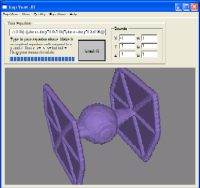# Imp ViewAn Implicit 3D Function Visualizer

Imp View lets you easily graph 3D implicit equations such as 'x^2 + y^2 + z^2 < 1' (the equation of a sphere). This lets you visualize many common equations without having to solve for them.

Note: - ImpView uses my VSaber Technology for interactive volumetric systems. VSaber is an amazing 3D modeling tool that 'is as easy to use as MS Paint'. ImpView uses the software renderer from VSaber, so that it will run on computers without great graphics cards.# Submitted Equations

 Johan Forsl�f sent in this really cool equation, the equation is below and it's graph is to the right. I'm sure anyone interested in an implicit equation grapher can appreciate this. (x^2+y^2+z^2<0.2)+((y^2+z^2<0.08)*(x<0.4)*(x>0))+(x^2+4*y^2<(1-abs z)*0.12)+((abs z<0.95)*(abs z>0.9)*(abs x+abs y*0.3<1))+((abs z<1)*(abs z>0.89))*((abs x<0.7)*(abs y>0.9)+(abs y<0.035)+(x>y*0.7-0.05)*(xy*0.7-0.05)*(-x0.95)))If you have an equation you would like to submit, just drop me a line at lewey@lewcid.com

To all you would be implicit models out there, Johan recomends using the C-preprocessor to generate 'complex' equation, as shown in an example below:

```#define CUT(a, b) (a) * (b)
#define SPHERE x^2 + y^2 + z^2 < 1
#define CYLINDER x^2 + y^2 < 1
CUT(SPHERE, CYLINDER)```

 ImpView.zipRequires: .Net Framework on WindowsUsage: Unzip and run. Make sure ImpViewer.dll is in the same folder as ImpView.exeNote: If it runs too slowly, turn the Quality down (in the 'Imp View' menu)

UPDATE - 11/10/2003 - ImpView just got a speed boost, and the ability to export the equation models thanks to the beta version of VSaber. You can now save your equation as a 3D model and edit it naturally using VSaber.

UPDATE - 9/26/2003 - After a couple hours making cool implicit equations (is it wrong to enjoy that?), I decided to add a few of the more interesting ones to the 'Equations' menu (these are a set of example equations), as well as fix a small math bug, give it a cool icon, and add a feature that lets you see 'inside' the volume instead of just it's surface (called 'View Inside Volume' in the 'View' menu). Oh and I reduced the default resolution down to 60^3 (change it in the 'Quality' menu). Enjoy.

Note: ImpView also includes a small symbolic equation evaluator. For stuff like 'sqrt(cos(pi^2))' or something. It's called 'Calc' under the 'Imp View' menu

Math Stuff: The system graphs the implicit equation by sampling it at discrete values in the given domain, if the function is true at that coordinate, it puts a point there (technically it puts a point if the result of the equation is non zero). However, don't try anything too fancy, I have only implimented the basic math operations (+ - * / ^ sin cos tan asin acos atan ln > >= < <= etc.). Also The reason I have you only enter equations with > >= < or <= in them is that if you used the equals sign, the result of the implicit equation would be a surface. And surfaces don't appear very well (or at all) when you discretely sample the surface like this system does.

Tech Stuff: I wrote this program in about 5 hours! Well sort of. Actually all I did was tie together a few parts from some of my other projects, and added a few extras like a fast evaluation core, interface, etc. First of all it uses the voxel engine I'm working on for the upcoming version of VSaber (only demo available right now). This engine is actually a full fledge volumetric carving system, here I just disabled a few of the editing features and used it as a visualizer. The rendering core of this engine is a high-performance software based algorithum, because it's targeted for Tablet PCs which don't have great graphics cards (which you need if your rendering 80^3 voxels like VSaber does. NOTE: 80^3 voxels is the default quality setting for Imp View). The expression parsing and scripting core come from Crystal Math, this let me convert a written equation into a set of steps which you could follow to evaluate that expression. I then wrote a high performance evaluator which let me very quickly see if the given equation is true at a certain (x,y,z) coordinate (and by true I mean non-zero, the < and > operators simply return 1 for true and 0 for false). Then simply bring the two together and voila!, you have can visualize a 3D implicit equation as a voxelized volume! Now I'm sure a few of you are thinking you could get better performance for volumetric rendering, this is very true. However those super fast rendering algorithms only work for static volumes, which makes them unsuitable for an interactive applications like VSaber. VSaber's engine is one of the few that uses a dynamic rendering system so that the volume can change from frame to frame. Why do I use it in this program then when the volume doesn't change that often? Because I didn't feel like writing another one when this one was just lying around!

Development: Imp View is a 'multi-lingual' application, but not in the usual sense. The voxel engine, and rendering core are written in C++ bordering on straight C. The scripting stuff was in C++. All of this was wrapped and made into a .Net Windows Control using Managed C++. The interface (buttons, text areas, etc.) were written in C#, using a DLL containing the Managed C++ voxel Control.